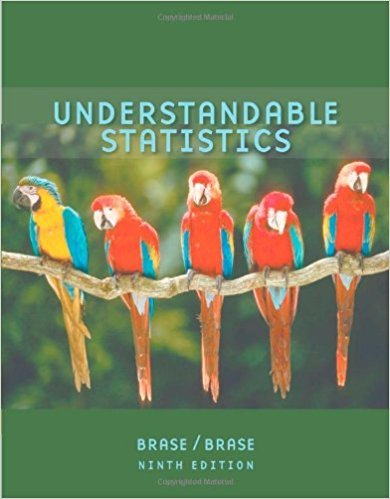×
×

# Statistical Literacy Consider a completely randomizedISBN: 9780618949922 213

## Solution for problem 2 Chapter 1.3

Understandable Statistics | 9th Edition

• Textbook Solutions
• 2901 Step-by-step solutions solved by professors and subject experts
• Get 24/7 help from StudySoup virtual teaching assistantsUnderstandable Statistics | 9th Edition

4 5 1 289 Reviews
27
0
Problem 2

Statistical Literacy Consider a completely randomized experiment in which a control group is given a placebo for congestion relief and a treatment group is given a new drug for congestion relief. Describe a double-blind procedure for this experiment and discuss some benefits of such a procedure.

Step-by-Step Solution:
Step 1 of 3

Statistics Notes Week of Oct. 24th 9.2: Hypothesis Test for Variables Forming a Null and Alternative Hypothesis Hypothesis Null Hypothesis:​ The conclusion that there is no correlation between the specified populations, meaning the populations are ​independent. ● The null hypothesis is written as Ho ● For example, if the sample is meant...

Step 2 of 3

Step 3 of 3

##### ISBN: 9780618949922

Understandable Statistics was written by and is associated to the ISBN: 9780618949922. The full step-by-step solution to problem: 2 from chapter: 1.3 was answered by , our top Statistics solution expert on 01/04/18, 09:09PM. The answer to “Statistical Literacy Consider a completely randomized experiment in which a control group is given a placebo for congestion relief and a treatment group is given a new drug for congestion relief. Describe a double-blind procedure for this experiment and discuss some benefits of such a procedure.” is broken down into a number of easy to follow steps, and 46 words. Since the solution to 2 from 1.3 chapter was answered, more than 241 students have viewed the full step-by-step answer. This full solution covers the following key subjects: . This expansive textbook survival guide covers 57 chapters, and 994 solutions. This textbook survival guide was created for the textbook: Understandable Statistics, edition: 9.

Unlock Textbook Solution